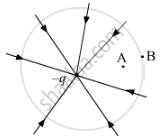# The Field Lines of a Negative Point Charge Are as Shown in the Figure. Does the Kinetic Energy of a Small Negative Charge Increase Or Decrease in Going from B to A? - Physics

The field lines of a negative point charge are as shown in the figure. Does the kinetic energy of a small negative charge increase or decrease in going from B to A?#### Solution

From the given field lines, we can say that the electric field present due to the given point charge will be directed towards the centre. Since we know that a negative charge always experiences a force in the direction opposite to that of the external electric field present, the negative charge will experience the force away from the centre. This will cause its motion to retard while moving from B to A. Hence, its kinetic energy will decrease in going from B to A.

Concept: Electric Field Due to a Point Charge
Is there an error in this question or solution?